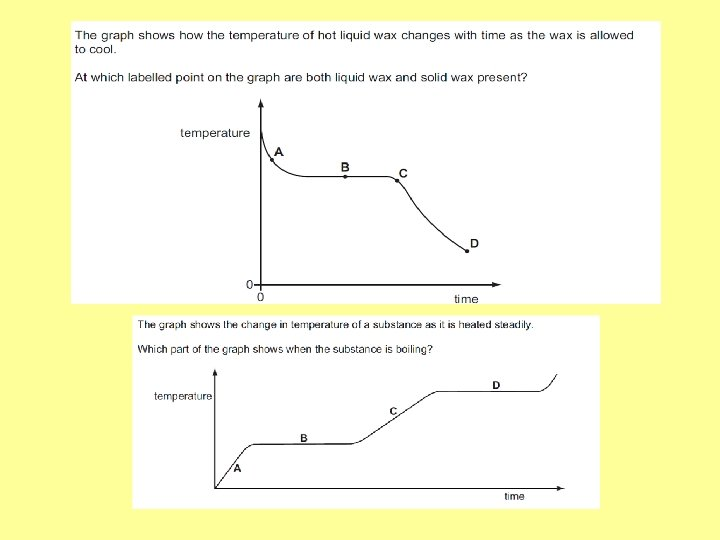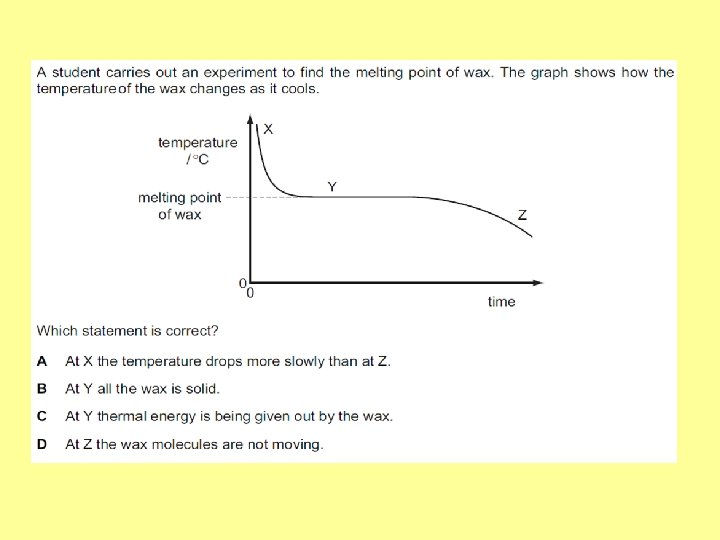# Latent Heat Boiling evaporating melting Gas Liquid Solid

• Slides: 15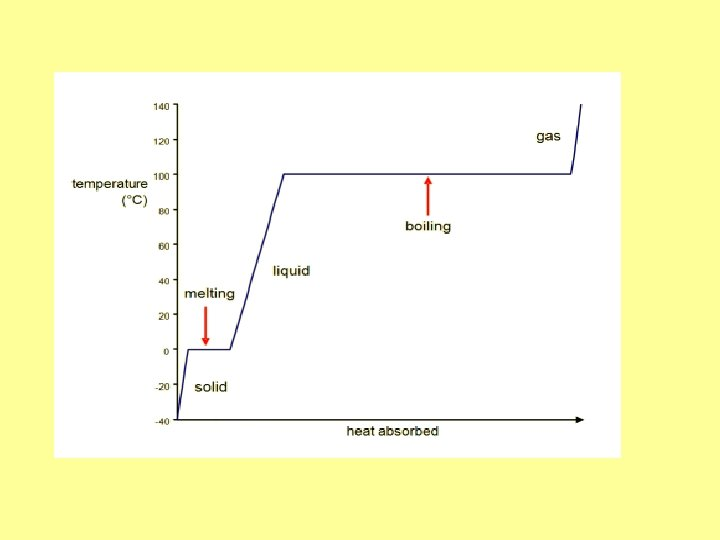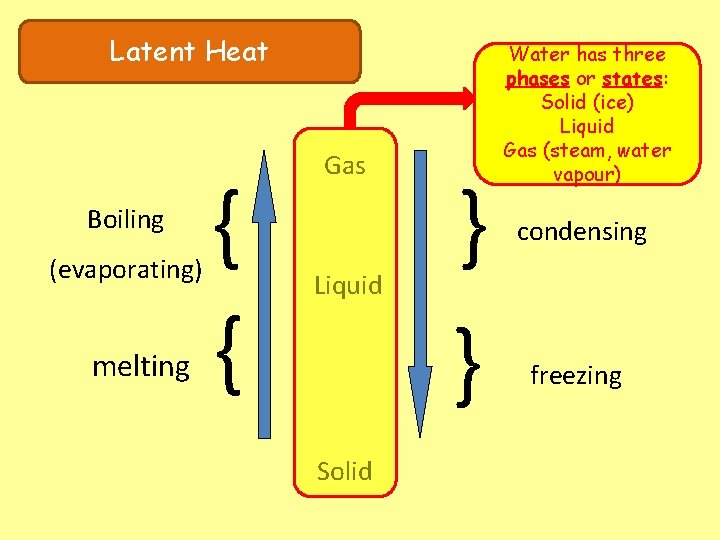Latent Heat Boiling (evaporating) melting { { Gas Liquid Solid } } Water has three phases or states: Solid (ice) Liquid Gas (steam, water vapour) condensing freezing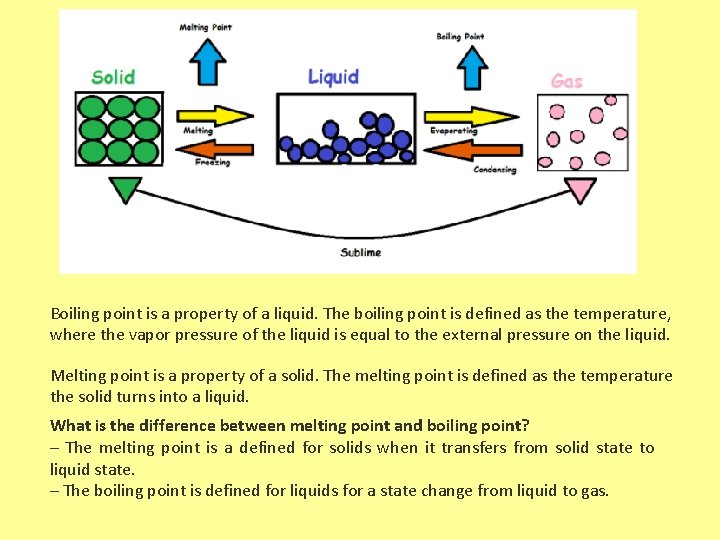Boiling point is a property of a liquid. The boiling point is defined as the temperature, where the vapor pressure of the liquid is equal to the external pressure on the liquid. Melting point is a property of a solid. The melting point is defined as the temperature the solid turns into a liquid. What is the difference between melting point and boiling point? – The melting point is a defined for solids when it transfers from solid state to liquid state. – The boiling point is defined for liquids for a state change from liquid to gas.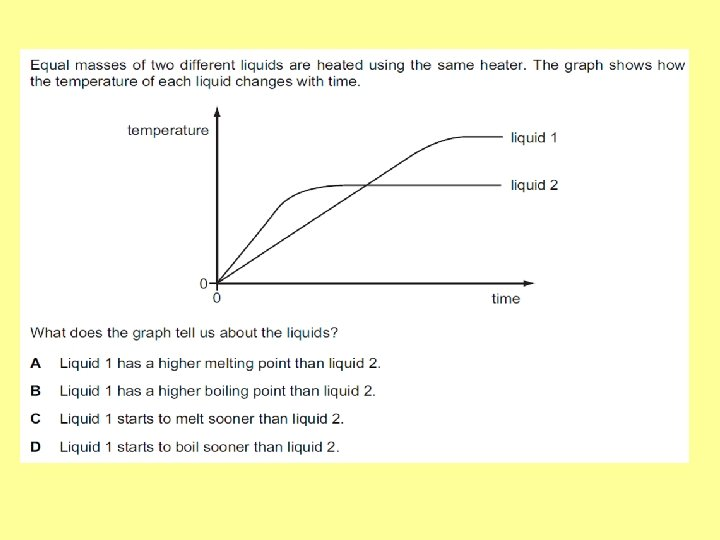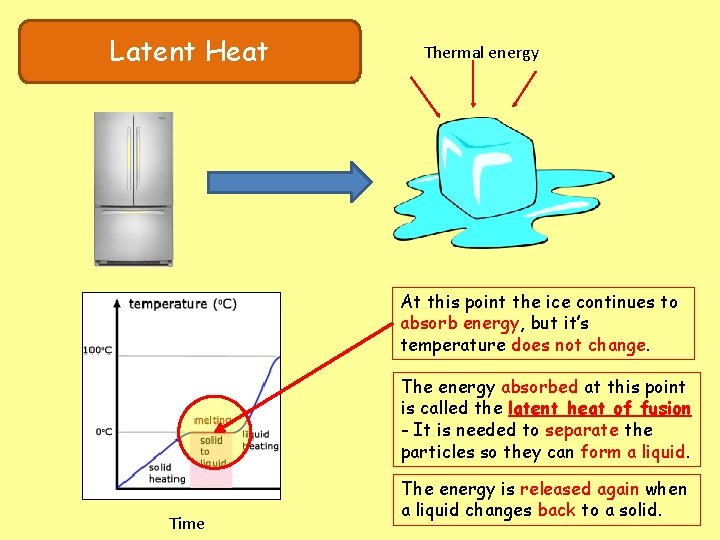Latent Heat Thermal energy At this point the ice continues to absorb energy, but it’s temperature does not change. The energy absorbed at this point is called the latent heat of fusion - It is needed to separate the particles so they can form a liquid. Time The energy is released again when a liquid changes back to a solid.Latent Heat eg. If 3. 5 kg of ice is melted (at 0 o. C) Q = m. L Energy transferred = 3. 5 x 330 000 The specific latent heat of fusion of ice is 330 000 J/kg Q = 1 155 000 J This means that 330 000 joules of energy are transferred to change each kilogram of ice into water at the same temperature (0 o. C). Equation: Energy transferred = mass x specific latent heat Q = m. LLatent Heat of Fusion Power = energy / time So, energy = Power x time Measuring the specific latent heat of fusion of ice. 100 W immersion heater switched on for 300 seconds. Q = 100 x 300 = 30 000 J Mass of water collected = 0. 10 kg Q = m. L L = Q/m L = 30 000 / 0. 10 = 300 000 J/kg Only an approximate figure for L as no allowance made for heat loss to the surroundings.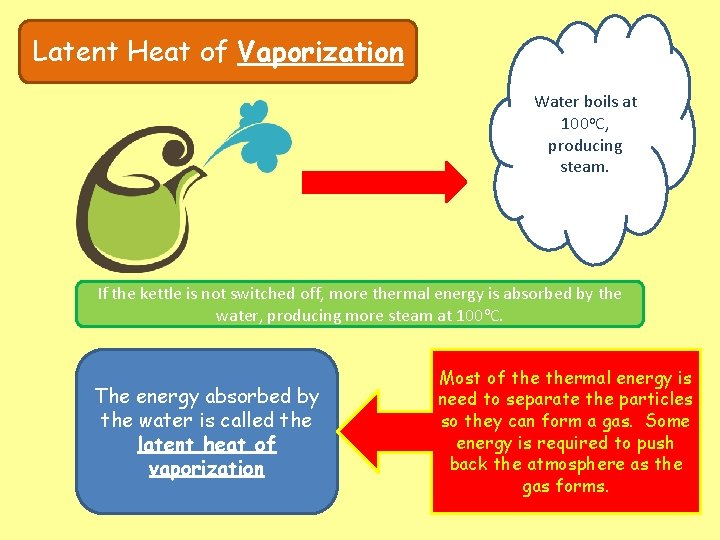Latent Heat of Vaporization Water boils at 100 o. C, producing steam. If the kettle is not switched off, more thermal energy is absorbed by the water, producing more steam at 100 o. C. The energy absorbed by the water is called the latent heat of vaporization Most of thermal energy is need to separate the particles so they can form a gas. Some energy is required to push back the atmosphere as the gas forms.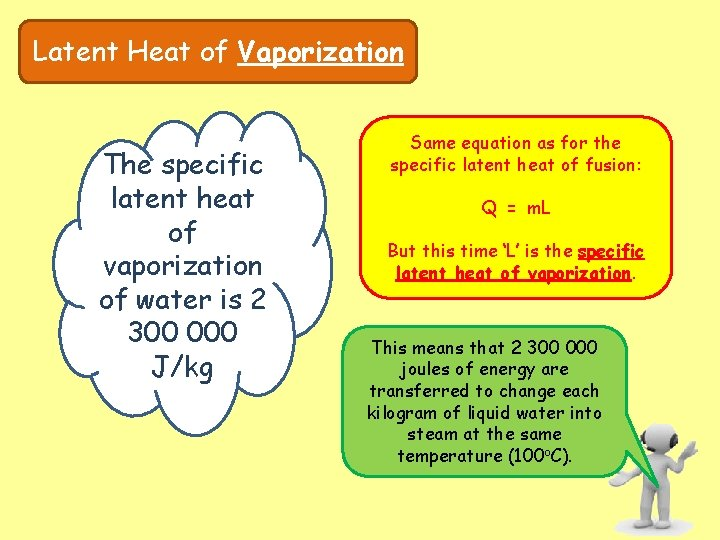Latent Heat of Vaporization The specific latent heat of vaporization of water is 2 300 000 J/kg Same equation as for the specific latent heat of fusion: Q = m. L But this time ‘L’ is the specific latent heat of vaporization. This means that 2 300 000 joules of energy are transferred to change each kilogram of liquid water into steam at the same temperature (100 o. C).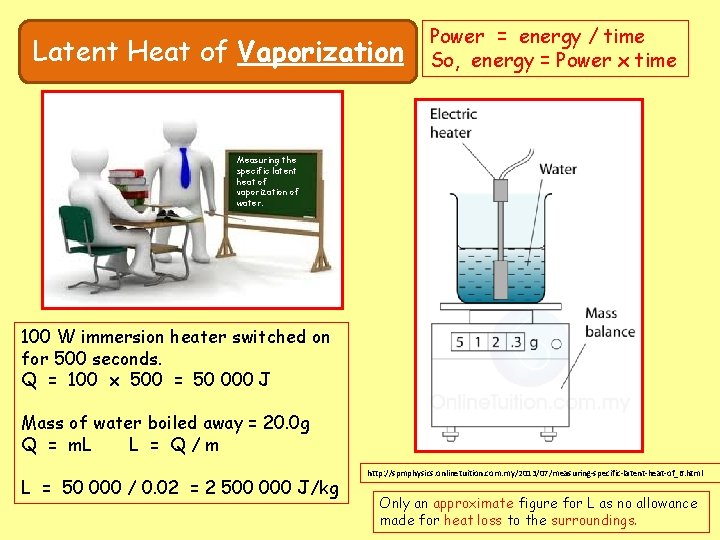Latent Heat of Vaporization Power = energy / time So, energy = Power x time Measuring the specific latent heat of vaporization of water. 100 W immersion heater switched on for 500 seconds. Q = 100 x 500 = 50 000 J Mass of water boiled away = 20. 0 g Q = m. L L = Q/m L = 50 000 / 0. 02 = 2 500 000 J/kg http: //spmphysics. onlinetuition. com. my/2013/07/measuring-specific-latent-heat-of_6. html Only an approximate figure for L as no allowance made for heat loss to the surroundings.